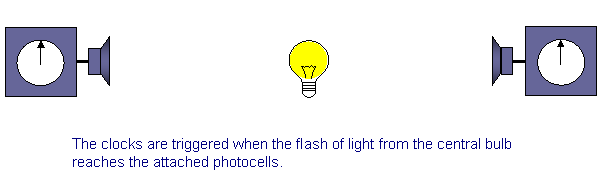# Special Relativity: Synchronizing Clocks

Michael Fowler, UVa Physics

Suppose we want to synchronize two clocks that are some distance apart.

We could stand beside one of them and look at the other through a telescope, but we’d have to remember in that case that we are seeing the clock as it was when the light left it, and correct accordingly.

Another way to be sure the clocks are synchronized, assuming they are both accurate, is to start them together. How can we do that? We could, for example, attach a photocell to each clock, so when a flash of light reaches the clock, it begins running.If, then, we place a flashbulb at the midpoint of the line joining the two clocks, and flash it, the light flash will take the same time to reach the two clocks, so they will start at the same time, and therefore be synchronized.

Let us now put this whole arrangement — the two clocks and the midpoint flashbulb — on a train, and we suppose the train is moving at some speed $v$ to the right, say half the speed of light or so.

Let’s look carefully at the clock-synchronizing operation as seen from the ground. In fact, an observer on the ground would say the clocks are not synchronized by this operation! The basic reason is that he would see the flash of light from the middle of the train traveling at $c$ relative to the ground in each direction, but he would also observe the back of the train coming at $v$ to meet the flash, whereas the front is moving at $v$ away from the bulb, so the light flash must go further to catch up.

In fact, it is not difficult to figure out how much later the flash reaches the front of the train compared with the back of the train, as viewed from the ground. First recall that as viewed from the ground the train has length $L 1− v 2 / c 2 .$Letting $t B$ be the time it takes the flash to reach the back of the train, it is clear from the figure that

$v t B +c t B = L 2 1− v 2 c 2$

from which $t B$ is given by

$t B = 1 c+v L 2 1− v 2 c 2 .$

In a similar way, the time for the flash of light to reach the front of the train is (as measured by a ground observer)

$t F = 1 c−v L 2 1− v 2 c 2 .$

Therefore the time difference between the starting of the two clocks, as seen from the ground, is

$t F − t B =( 1 c−v − 1 c+v ) L 2 1− ( v/c ) 2 =( 2v c 2 − v 2 ) L 2 1− ( v/c ) 2 = 2v c 2 1 1− ( v/c ) 2 L 2 1− ( v/c ) 2 = vL c 2 1 1− ( v/c ) 2 .$

Remember, this is the time difference between the starting of the train’s back clock and its front clock as measured by an observer on the ground with clocks on the ground.  However, to this observer the clocks on the train appear to tick more slowly, by the factor $1− v 2 / c 2 ,$ so that although the ground observer measures the time interval between the starting of the clock at the back of the train and the clock at the front as

$( vL/ c 2 )⋅ 1 1− v 2 / c 2$ seconds, he also sees the slow running clock at the back actually reading $vL/ c 2$ seconds at the instant he sees the front clock to start.

To summarize: as seen from the ground, the two clocks on the train (which is moving at $v$ in the $x$ -direction) are running slowly, registering only $1− v 2 / c 2$ seconds for each second that passes.  Equally important, the clocks$—$which are synchronized by an observer on the train$—$appear unsynchronized when viewed from the ground, the one at the back of the train reading $vL/ c 2$ seconds ahead of the clock at the front of the train, where $L$ is the rest length of the train (the length as measured by an observer on the train).

Note that if $L=0,$ that is, if the clocks are together, both the observers on the train and those on the ground will agree that they are synchronized.  We need a distance between the clocks, as well as relative motion, to get a disagreement about synchronization.